# Nbody6tid Manual

Andreas Ernst
Astronomisches Rechen-Institut am
Zentrum für Astronomie der Universität Heidelberg,
Mönchhofstr. 12-14, 69121 Heidelberg, Germany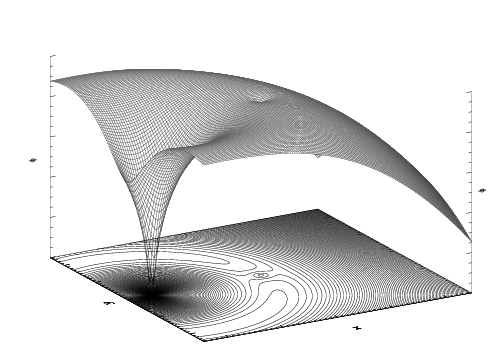Version 06/2012

### 1 Preface

This manual is intended to be a documentation for students and postdocs of the three variants NBODY6TID, NBODY6TIDPAR and NBODY6TIDGPU of Sverre Aarseth’s direct N-body code NBODY6. The author would like to note that that the name NBODY6TID and the first simple version of this program stem from Rainer Spurzem. For a documentation of NBODY6 the reader is first and foremost referred to the webpage of Sverre Aarseth (http://www.ast.cam.ac.uk/~sverre/) which contains a code repository as well as documentation and to the manual NBODY6++ - Features of the computer-code by Emil Khalisi and Rainer Spurzem. Only if the reader is interested to solve the N-body problem in the tidal field of a galaxy such as the Milky Way (which can be represented as a 3-component Plummer-Kuzmin model with special parameters), that of a galactic center (with a power-law gravitational potential) or in other special tidal fields like that of a Plummer or Dehnen model, this manual may be useful to him.

Heidelberg, June 2012
Andreas Ernst

### 2 Introduction

The programs NBODY6TID, NBODY6TIDPAR and NBODY6TIDGPU are three variants of the direct N-body code NBODY6 by Sverre Aarseth (Aarseth 1999, 2003). They integrate the N-body problem in an analytic background potential of a galaxy or a galactic centre.

• NBODY6TID is the standard serial code which runs on single processors.
• NBODY6TIDPAR runs on parallel machines (ranging from small Beowulf clusters up to large supercomputers) using the Message Passing Interface (MPI) for communication between the processors. This variant is based on NBODY6++ (Spurzem 1999).
• NBODY6TIDGPU runs on multicore processors (via OpenMP) with one or two Graphics Processing Units (GPUs). For this purpose, special routines in CUDA have been added by Keigo Nitadori in collaboration with Sverre Aarseth. The variant of NBODY6 with GPU support was termed NBODY6GPU (Nitadori & Aarseth 2011).

The special feature of NBODY6TID, NBODY6TIDPAR and NBODY6TIDGPU is the implementation of different tidal fields. This implementation is based on the implementation in the (parallel) code NBODY6GC which is described in detail in Ernst, Just & Spurzem (2009) and Ernst (2009). Several analytic models for the background potential are now implemented, e.g.,

• power-law models of the gravitational potential with and without a central black hole
• Plummer model (Plummer 1911).
• Dehnen models (Dehnen 1993, Tremaine et al. 1994)
• three-component Plummer-Kuzmin model of the Milky Way (Miyamoto & Nagai 1975)

In addition to the equations of motion of the N-body problem, NBODY6TID solves the equations of motion of the star cluster orbit around the Galactic center with an eighth-order composition scheme (Yoshida 1990; for the coefficients see McLachlan 1995). The tidal force of the background potential acts on all particles in the N-body system and is added as a perturbation to the KS regularization (Kustaanheimo & Stiefel 1965) of NBODY6.

NBODY6TID is able to integrate star cluster orbits with almost all eccentricities. For nearly radial orbits a time transformation can be switched on to guarantee an exact orbit integration during the pericenter passages. The leapfrog-like symplectic integration schemes allow for adaptive time steps if one applies a Sundman transformation to the time (Sundman 1912, Mikkola & Tanikawa 1999, Preto & Tremaine 1999, Mikkola & Aarseth 2002).

It is also possible to switch on a semianalytical treatment of Chandrasekhar’s dynamical friction with different χ functions and different realizations of the Coulomb logarithm, i.e. fixed or variable according to Just & Peñarrubia (2005). Since the symplectic composition schemes are by construction suited to Hamiltonian systems, the dissipative dynamical friction force requires special attention. It is implemented in NBODY6TID with an implicit midpoint method using an iteration (see, e.g., Mikkola & Aarseth 2002).

### 3 Tidal fields

The tidal field (or effective tidal potential) is given by the superposition of the galactic gravitational potential and the centrifugal potential, i.e. we usually have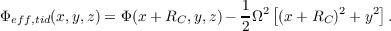(1)

where RC is the radius of the circular orbit. For a circular orbit, the tidal field resembles a ring-like parabolic (to second order) ridge around the galactic center (see figure on the title page). In this ridge the star cluster potential is embedded. For a spherically symmetric star cluster potential the equilibrium Lagrange points L1 - L5 naturally arise. Therefore the total gravitational potential is given by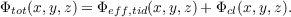(2)

Here the origin is shifted to the star cluster center at (x,y,z) = (0,0,0). For the star cluster potential, a Plummer model can be inserted.

#### 3.1 Spherically symmetric models

We define r =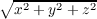for the spherically symmetric models, where x,y andd z are Cartesian coordinates. G is the gravitational constant and M a mass. The velocity components are vx,vy and vz.

##### 3.1.1 Kepler potential

The Kepler potential is given by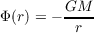(3)

The acceleration is given by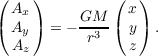(4)

The jerk is given by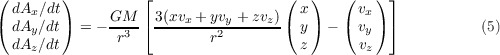##### 3.1.2 Power-law models

The spherically symmetric power-law or scale-free models have a potential of the form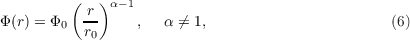where r is the radius and r0 is a length unit. The acceleration has the form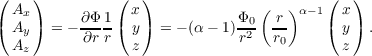(7)

The jerk is given by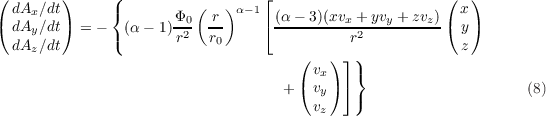A supermassive black hole can be added to the center of such a model.

##### 3.1.3 Plummer model

The gravitational potential of a Plummer model (Plummer 1911) is given by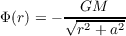(9)

where a is the Plummer radius. The acceleration is then given by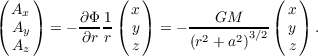(10)

The jerk is given by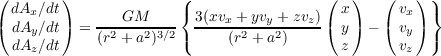(11)

##### 3.1.4 Dehnen model

The gravitational potential of a Dehnen model (Dehnen 1993, Tremaine et al. 1994) is given by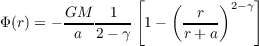(12)

where a is a scale radius. The acceleration is then given by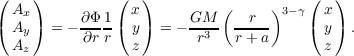(13)

The jerk is given by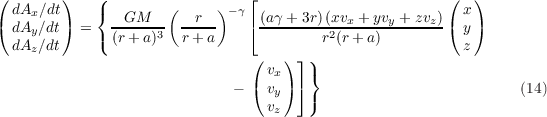##### 3.1.5 Logarithmic potential

A logarithmic gravitational potential is given by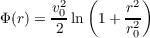(15)

The acceleration is given by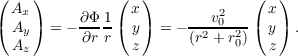(16)

The jerk is given by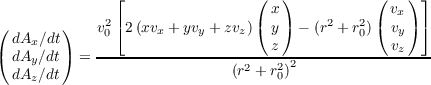(17)

#### 3.2 Axisymmetric three-component models

##### 3.2.1 3PLK Milky Way model

Table 1: The list of galaxy component parameters for the 3PLK model of the Milky Way (Peter Berczik, Patrick Glaschke).

 Component M [M⊙] a [kpc] b [kpc] Bulge 1.4 × 1010 0.0 0.3 Disk 9.0 × 1010 3.3 0.3 Halo 7.0 × 1011 0.0 25.0

Table 2: Circular velocities at different galactocentric radii (at z = 0) for the 3PLK model with the parameters of table 1. The astronomical constants are taken from Binney & Tremaine (2008), appendix A.

 Rg [kpc] V cir [km s-1] Rg [kpc] V cir [km s-1] 0.5 279.269858420500 10.5 231.472619038406 1.0 246.015548276821 11.0 231.416801583084 1.5 230.132164798490 11.5 231.499317939399 2.0 228.141259503200 12.0 231.704811326295 2.5 231.286020479153 12.5 232.017589382089 3.0 235.208140475138 13.0 232.422164626838 3.5 238.261145511816 13.5 232.903626883944 4.0 240.070687448505 14.0 233.447890039686 4.5 240.763498292986 14.5 234.041845222914 5.0 240.613878677284 15.0 234.673444774045 5.5 239.899715058196 15.5 235.331735556181 6.0 238.853489734555 16.0 236.006855744588 6.5 237.652904859520 16.5 236.690005856049 7.0 236.426154512127 17.0 237.373402187912 7.5 235.261261678691 17.5 238.050218839306 8.0 234.215362687272 18.0 238.714522944716 8.5 233.322594742001 18.5 239.361206558702 9.0 232.600357491854 19.0 239.985917711179 9.5 232.054117466732 19.5 240.584992445018 10.0 231.681026811365 20.0 241.155389105121

Table 3: The value of β = κ∕Ω at different galactocentric radii (at z = 0) for the 3PLK model with the parameters of table 1. κ and Ω are the epicyclic and circular frequencies, respectively, of an orbit which is close to be circular. The astronomical constants are taken from Binney & Tremaine (2008), appendix A.

 Rg [kpc] β Rg [kpc] β 0.5 1.3605 10.5 1.4059 1.0 1.2520 11.0 1.4153 1.5 1.3474 11.5 1.4245 2.0 1.4342 12.0 1.4333 2.5 1.4737 12.5 1.4415 3.0 1.4781 13.0 1.4490 3.5 1.4639 13.5 1.4558 4.0 1.4425 14.0 1.4619 4.5 1.4201 14.5 1.4671 5.0 1.4002 15.0 1.4715 5.5 1.3843 15.5 1.4751 6.0 1.3728 16.0 1.4780 6.5 1.3658 16.5 1.4802 7.0 1.3627 17.0 1.4816 7.5 1.3631 17.5 1.4824 8.0 1.3664 18.0 1.4825 8.5 1.3719 18.5 1.4821 9.0 1.3791 19.0 1.4812 9.5 1.3874 19.5 1.4798 10.0 1.3965 20.0 1.4779

The parameters of the 3-component Plummer-Kuzmin model (Miyamoto & Nagai 1975) of the Milky Way are given in table 1 or Just et al. (2009). The total gravitational potential is given by a a superposition of three potentials for bulge, disk and halo. A few quantities as a function of galactocentric radius are given in tables 2 and 3. The gravitational potential has the form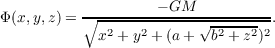(18)

The acceleration for the Plummer-Kuzmin model is given by the expression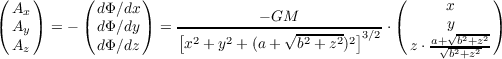(19)

The jerk is given by the lengthy expressions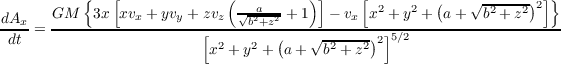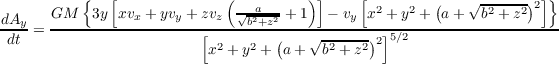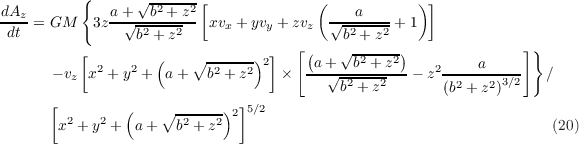which also depend on the velocity components.

##### 3.2.2 JSH95 Milky Way model (not yet implemented)

The gravitational potential of the JSH95 model (see Dinescu et al. 1999) is given by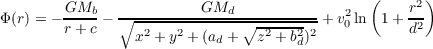(21)

with Mb = 3.4 × 1010M, c = 0.7 kpc, Md = 1011M, ad = 6.5 kpc, bd = 0.26 kpc, v0 = 128 km s-1, d = 12.0 kpc. The bulge has a Hernquist profile, the disk is represented as a Plummer-Kuzmin model and the halo by a logarithmic potential.

The acceleration for the JSH95 model is given by the expression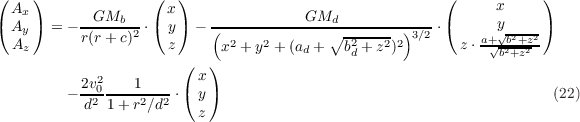##### 3.2.3 P90 Milky Way model (not yet implemented)

The gravitational potential of the P90 model (see Dinescu et al. 1999) is given by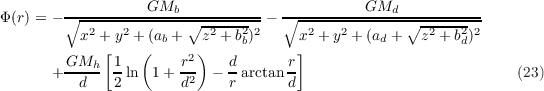with Mb = 1.12 × 1010M, ab = 0.0 kpc, bb = 0.277 kpc, Md = 8.0710M, ad = 3.7 kpc, bd = 0.20 kpc, Mh = 5 × 1010M, d = 6.0 kpc.

The acceleration for the P90 model is given by the expression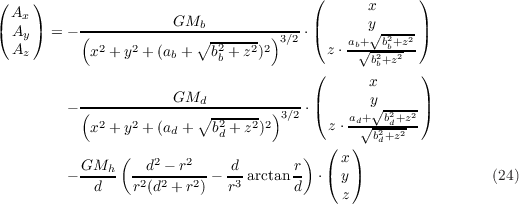### 4 New Subroutines and code changes

#### 4.1 New subroutines

All new subroutines in NBODY6TID begin with the letters “EXT” for “EXTernal tidal field” or “EXTernal galaxy”. Table 4 gives an overview of the routines with a short description.

 Subroutine Description Called by subroutine EXTINIT GC initializations START EXTGALAX GC external force on star cluster REGINT, KSPERT EXTGALAX2 Calculates all galaxy quantities OUTPUT, EXTINIT, EXTINT, EXTCHECK, EXTMASS, EXTCHI, EXTXCOUL EXTFORCE Calculate GC force EXTINT, EXTGALAX EXTFPR Time transformation (optional) EXTINIT, EXTINT EXTINT GC integrator INTGRT EXTCHECK GC energy check ADJUST, OUTPUT EXTENERGY Calculate GC energy EXTCHECK, EXTINIT, EXTINT, EXTMASS EXTMASS Star cluster mass and members EXTINIT, EXTINT EXTOUTP GC data output OUTPUT EXTIPOL Interpolation of GC jerk if not known EXTINT EXTCHI Calculates current value of χ EXTINT, EXTOUTP EXTXCOUL Calculates current value of lnΛ EXTINIT, EXTOUTP

Table 4: New subroutines of NBODY6TID. “GC” stands for galactic center. The “design” of the table is by Patrick Glaschke.

#### 4.2 Changes to nbody6/nbody6++

The following changes have been made to the original program NBODY6:

• The subroutines shown in table 4 have been added as well as the calls of these subroutines.
• The option KZ(14)=5 is used for NBODY6TID.
• A new common block /EXT/ has been added to common6.h. The routine mydump.f has been adjusted accordingly.
• The array AS(20) in the routine output.f has been extended to AS(30) with more entries which include a few parameters of the external tidal field, in particular the position and velocity of the galactic center particle.
• ...

### 5 Input file: galax.dat

Shown below are two examples of the additional input file called galax.dat which contains the parameters of the external tidal field.

#### 5.1 Example for the 3PLK model

0.001 0.0 1.0 8.0 3.0 0 0
7 9 2 0 0.0 0.0
1.4e10 0.0 0.3 9.0e10 3.3 0.3 7.0e11 0.0 25.0
-8000.0 0.0 0.0 0.0 234.211656579 0.0

ETAG,GAMMA,VCIRC,RGAL,XTIDAL,ICLUST, IENRG
NGALAX,NCB,NCHI,VARI,XCOUL,QFIT
CB(K)
XG(1) XG(2) XG(3) VG(1) VG(2) VG(3)

#### 5.2 Example for a power-law model in the Galactic center

1.0 0.0 1.0 10.0 2.0 2 1
0 7 1 0 0.0 1.0
0.589048 100. 0. 1.2 0.1 4.6 3.6

ETAG,GAMMA,VCIRC,RGAL,XTIDAL,ICLUST, IENRG
NGALAX,NCB,NCHI,VARI,XCOUL,QFIT
CB(K)

#### 5.3 Input variables

The input variables have the following purpose:

• ETAG: Accuracy parameter of galactic center intgration, similar to ETAI, ETAR in NBODY6 (currently recommendable are values between 0.001 and 1.0. A good estimate for the Milky Way is ETAG=(0.1 RGAL [pc])-1)
• GAMMA: Potential energy exponent for time transformation (=0.0: constant time steps; -1.0, -2.0, -3.0, ...: adaptive time steps for eccentric orbits according to Preto & Tremaine 1999, eq. 26)
• VCIRC: Tangential cluster velocity in units of circular velocity at cluster position (=1.0: circular orbit, 0.0 < VCIRC < 1.0: eccentric orbit; not used for the 3PLK model; here the fourth row of galax.dat is used)
• RGAL: Distance of cluster from galactic center in units of the virial cluster radius (not used for the 3PLK model; here the fourth row of galax.dat is used)
• XTIDAL: XTIDAL times the Jacobi radius is assumed as spherical cluster boundary
• ICLUST: Select how to treat cluster mass (=0: constant mass; =1: tidal radius approximation using XTIDAL; =2: with tidal radius and dynamical friction only for cluster members)
• IENRG: Energy conservation treatment (=0: approximation by Aarseth/Heggie for weak tidal fields; =1: exact for strong tidal fields)
• NGALAX: Galaxy model (see model-par.txt)
• NCB: Number of CB(K) parameters to be read in
• NCHI: Type of chi function for dynamical friction (see model-par.txt)
• VARI: Type of variable Coulomb logarithm (see model-par.txt)
• XCOUL: Coulomb logarithm (=0.0: No dynamical friction)
• QFIT: Fitting factor for Coulomb Logarithm (see Just & Penarrubia 2005)
• CB(K): External galaxy parameters (see chapter 6)
• XG(1), XG(2), XG(3), VG(1), VG(2), VG(3): Galactic center position and velocity in pc and km/s for the 3PLK model

### 6 Parameter settings

• NGALAX: Type of galaxy

0
Scale free with black hole
1
Kepler
2
Logarithmic potential
3
Isotermal with core and cutoff
4
Dehnen model
5
Plummer model
6
Scale free without black hole
7
3-component Plummer-Kuzmin model

• NCHI: Type of χ function
0
from file chi(v,phi) (e.g. Dehnen models)
1
Maxwell with 2σ2 = V cir2
2
King model (not yet implemented)
3
Plummer model

• VARI: Type of Coulomb logarithm
0
fixed Coulomb logarithm
1
linearly increasing during first orbit?
2
variable Coulomb logarithm (Just & Penarrubia 2005)

• CB(K): Galaxy parameter in N-body units
1
rcore (isothermal), a (Dehnen, Plummer), MBulge (3PLK)
2
rcut (isothermal), aBulge (3PLK)
3
σ0 (isothermal), bBulge (3PLK)
4
Power index for galactic bulge mass profile (Scale free), MDisk (3PLK), γ (Dehnen)
5
6
Galactic bulge mass at 1 pc (Scale free), bDisk (3PLK) Total mass in units of star cluster mass (Dehnen, Plummer, isothermal)
7
Black hole mass (Scale free, Kepler), MHalo (3PLK)
8
aHalo (3PLK)
9
bHalo (3PLK)

### 7 Planning of a run

For the planning of a new run or even a parameter space you should invest some time. To setup a run, you need to choose the parameter of the star cluster and the galaxy in which the star cluster is orbiting, the parameters of the orbit (i.e., the eccentricity and positioning of the orbit, should dynamical friction be turned on?). Then you must change the input files in and galax.dat accordingly. It is always useful to calculate also the relevant time scales. Let us define that rV and tV are the N-body units of length and time. We have rV = RBAR pc. Then the setup of a run proceeds as follows:

1.
Choose star cluster parameters

(a)
Particle number N
(b)
Type of model (King, Plummer) rh∕rV [see Table 1 in Gürkan, Freitag & Rasio (2004)]
(c)
Half-mass radius rh [pc] rV [pc], RBAR
(d)
Star cluster mass Mcl [M] ZMBAR
2.
Choose galaxy parameters

(a)
Type of model (Scale free, Dehnen, Kepler, Plummer, Plummer-Kuzmin)
(b)
CB constants (see chapter 6, note that some constants depend on Mcl!)
3.
Choose dynamical friction treatment

(a)
Fixed / Variable Coulomb logarithm / Vanishing Coulomb logarithm
(b)
Type of χ function
4.
Choose initial conditions of star cluster orbit

(a)
Initial galactocentric radius Rg(0) [rV ] or position Xg,Y g,Zg
(b)
Initial velocity V g(0) [V c] or velocity V g,x,V g,y,V g,z
5.
Determine time scales

(a)
N-body time unit tV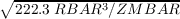Myr
(b)
Orbital time scale of star cluster torb (use INTEXT)
(c)
Half-mass relaxation time trh 0.192N∕ln(γN)tV
(d)
Crossing time inside the star cluster tcr = 2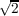tV

### 8 Starting the code

If you want to use the code, you need to compile it first. The current standard Fortran 77 compiler is gfortran, but you may use ifort or pgf77 as well. However, you must change the Makefile, Makefile_gpu and Makefile_sse appropriately. For the GPU version you need a machine which is equipped with a GPU. For the parallel version you need access to a parallel cluster or a supercomputer. The main routines of the standard serial code are in the folder /Ncode, the GPU routines are in the folder /GPU and its subfolder /GPU/lib. The stand-alone version of the parallel code is located in the folder /PAR. In the subfolders /GPU/run and /PAR/run are examples of the input files in, inmod (the restart input file) and galax.dat for the 3-component Plummer-Kuzmin (3PLK) model and the scale-free (SCF) model. Important: The variable KZ(14) must be set to the value KZ(14)=5 in the standard input file of NBODY6.

#### 8.1 Starting nbody6tid

cd nb6tid_BETA
cd Ncode
make clean
make

cd ..
mkdir run
cp Ncode/nbody6 run
cp GPU/run/in run
cp GPU/run/galax.dat run
cd run
./nbody6 < in > out 2>&1 &
tail -f out

#### 8.2 Starting nbody6tidpar

cd nb6tid_BETA
cd PAR
make clean
make mpich

...

The following commands depend on the specific architecture of the parallel cluster or supercomputer. When you are submitting a job to a small Beowulf cluster, you typically have to use a queue submission command like qsub within a console. When you are submitting to a large supercomputer, you may have to make use of a submission client like UNICORE with a comfortable graphical user interface. For GRID jobs you may also have to use the console-based GLOBUS toolkit.

#### 8.3 Starting nbody6tidgpu

cd nb6tid_BETA
cd Ncode
make clean
cd ..
cd GPU
make clean
make gpu

cd run
./nbody6.gpu < in > out 2>&1 &
tail -f out

#### 8.4 Scripts

In the run folder there are backup scripts called backup.sh and restore.sh. They can be used if a run has stopped for some reason and will be restarted several times. The backup script tests whether a backup already exists. Usage:

./backup.sh <number>

./restore.sh <number>

The important output files of nbody6 are backed up under a number and can be restored.

There is also a script called clean.sh which cleans up the run directory:

./clean.sh

#### 8.5 Snapshots

The programs “snap.f” (and “snaprho.f”) calculate a snapshot at a certain time in the format

m x y z vx vy vz (rho)

For the calculation of the density the procedure described in Casertano & Hut (1985) is applied. The number of neighbors (e.g. Nnb = 5 - 20) is a free parameter.

### 9 Data output

The additional data output of NBODY6TID has the following form. You may type something like

grep EXTPOS out > extpos.dat

to obtain a file with the coordinates of the galactic center.

#### 9.1 Energy data output

- EXT check ------------------------------------------------------------------------------------------------------------------------------------------------------------------------------------------------
EXTCHECK1: T=       1.00   EG0=  -0.15797073E+06   DEDF1=   0.31556875E+05   DEDF2=  -0.92853539E-04   DEDF3=  -0.18952760E+06   DEDFF=   0.00000000E+00   DEML2=   0.00000000E+00   ETIDE=   0.24983997E-04
EXTCHECK2: T=       1.00   EBIN=  -0.4696E-04   ZKIN=     0.2664   -POT=      -0.5163   ETIDE=   0.2498E-04   ECORR=   0.0000E+00   ETOT=    -0.2500
EXTCHECK3: T=       1.00   EGIN=  -0.1580E+06   EGCUR=  -0.1580E+06   EGKIN=   0.3156E+05   EGPOT=  -0.1895E+06   DEFRIC=   0.0000E+00   DEMALO=   0.0000E+00
EXTCHECK4: T=       1.00   EGCUR=  -0.1580E+06   DEFRIC=   0.0000E+00   DEMALO=   0.0000E+00   DECORR=   0.0000E+00   DEG=  -0.2910E-09   DEEG=   0.1842E-14
EXTCHECK5: T=       1.00   ESUB=   0.0000E+00   EMERGE=   0.0000E+00   ECOLL=   0.0000E+00   EMDOT=   0.0000E+00   ECDOT=   0.0000E+00
------------------------------------------------------------------------------------------------------------------------------------------------------------------------------------------------------------

#### 9.2 Tidal field data output

- EXT output --------------------------------------------------------------------------------------------------------------------------------------------------
EXTPOS:     T=       1.00   XG=   -8496.2245    252.9654      0.0000   VG=       7.4974    251.1126      0.0000   RDENS=      -0.0185     -0.0085      0.0352
EXTGAL:     T=       1.00   RHO=   0.5623E-03   MR=   0.0000E+00   POT=  -0.1895E+06   VCIR=   0.0000E+00
EXTFRIC:    T=       1.00   RGAL=    8499.9895   RHO=   0.5623E-03   CHI=                  NaN   LNL=    0.000000000000000
EXTINT:     T=       1.00   DTCEN=   0.2829E-02
EXTTIDE:    T=       1.00   RGAL=    8499.9895   XMC=   1.0000   RTIDAL=   0.1893E+01   RTPHYS=   0.8131E+01   NMEM=     3000
EXTCMR:     T=       1.00   CMR=  -0.1332E-06 -0.2842E-06 -0.3355E-06   CMRDOT=  -0.2422E-06 -0.3625E-06 -0.3656E-06
--------------------------------------------------------------------------------------------------------------------------------------------------------------

### 10 Simple integrator: IntExt

The folder “/IntExt” contains a simple integrator called INTEXT (“INTegrate EXTernal galaxy”) for star cluster orbits in the tidal field.1 The only difference to NBODY6TID is that the N-body problem is not solved. Therefore the integration is very fast.

Usage: Adapt galax.dat input file to your needs.2 Then start the program as follows:

cd IntExt
make clean
make
./intext

At the end of the integration the relative energy error is printed out. Make sure that it is sufficiently small.

### 11 Acknowledgements

Thanks go to Dr. Sverre Aarseth, Prof. Dr. Rainer Spurzem, Dr. Keigo Nitadori, Dr. Peter Berczik, Dr. Andreas Just, Prof. Dr. Ortwin Gerhard, Dr. Kap Soo Oh and Dr. Godehard Sutmann. Further thanks go to those who contributed to NBODY6TID through discussions, mainly the members of the stellar dynamics group at ARI.

### References

   Aarseth S. J., 1999, PASP, 111, 1333

   Aarseth S. J., 2003, Gravitational N-body simulations - Tools and Algorithms, Cambridge Univ. Press, Cambridge, UK

   Binney J., Tremaine S., Galactic Dynamics, 2008, 2nd ed., Princeton Univ. Press, Princeton, USA

   Casertano S., Hut P., 1985, Ap. J., 298, 80

   Dehnen W., 1993, MNRAS, 265, 250

   Dinescu D.I., Girard T. M., van Altena W. F., 1999, AJ, 117, 1792

   Ernst A., 2009, Dissolution of star clusters in the Galaxy and its Center, PhD thesis, Univ. of Heidelberg, Germany, http://www.ub.uni-heidelberg.de/archiv/9375

   Ernst A., Just A., Spurzem R., 2009, MNRAS 399, 141

   Gürkan M. A., Freitag M., Rasio F. A., 2004, Ap. J., 604, 632

   Just A., Peñarrubia J., 2005, A&A, 431, 861

   Just A., Berczik P., Petrov M.I., Ernst A., 2009, MNRAS, 392, 969

   Kustaanheimo P. Stiefel E. L., 1965, J. für reine angewandte Mathematik, 218, 204

   McLachlan R., 1995, SIAM J. Sci. Comp., 16, 151

   Mikkola S., Tanikawa K., 1999, Cel. Mech. Dyn. Astron., 74, 287

   Mikkola S., Aarseth S. J., 2002, Cel. Mech. Dyn. Astron., 84, 343

   Miyamoto M., Nagai R., 1975, PASJ 27, 533

   Nitadori K., Aarseth S. J., 2011, MNRAS, submitted

   Plummer H. C. 1911, MNRAS 71, 460

   Preto M., Tremaine S. 1999, AJ, 118, 2532

   Spurzem R., 1999, J. Comp. Appl. Maths, 109, 407

   Sundman K. F., 1912, Acta Math., 36, 105

   Tremaine S. et al., 1994, AJ, 107, 634

   Yoshida H., 1990, Phys. Lett. A, 150, 262

### A To Do’s

• Implement JSH95 and P90 models
• Implement V cir,M(R)(R) for all models
• Exact energy conservation SN kicks (IENRG=1): A call of EXTCHECK in INTGRT shows, that ETIDE changes on a short time scale (1 time unit) The reason is probably the mixed term VG*XDOT?
• Test exact energy conservation stellar evolution mass loss (IENRG=1)
• Test runs with primordial binaries